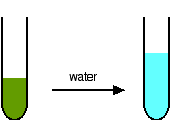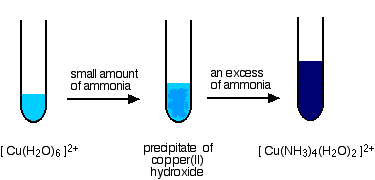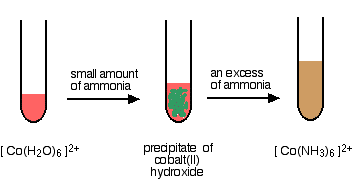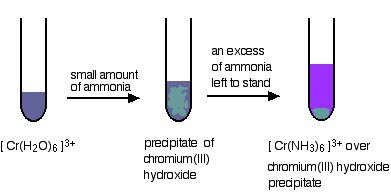# Ligand Exchange Reactions (Introduction)

$$\newcommand{\vecs}{\overset { \rightharpoonup} {\mathbf{#1}} }$$ $$\newcommand{\vecd}{\overset{-\!-\!\rightharpoonup}{\vphantom{a}\smash {#1}}}$$$$\newcommand{\id}{\mathrm{id}}$$ $$\newcommand{\Span}{\mathrm{span}}$$ $$\newcommand{\kernel}{\mathrm{null}\,}$$ $$\newcommand{\range}{\mathrm{range}\,}$$ $$\newcommand{\RealPart}{\mathrm{Re}}$$ $$\newcommand{\ImaginaryPart}{\mathrm{Im}}$$ $$\newcommand{\Argument}{\mathrm{Arg}}$$ $$\newcommand{\norm}{\| #1 \|}$$ $$\newcommand{\inner}{\langle #1, #2 \rangle}$$ $$\newcommand{\Span}{\mathrm{span}}$$ $$\newcommand{\id}{\mathrm{id}}$$ $$\newcommand{\Span}{\mathrm{span}}$$ $$\newcommand{\kernel}{\mathrm{null}\,}$$ $$\newcommand{\range}{\mathrm{range}\,}$$ $$\newcommand{\RealPart}{\mathrm{Re}}$$ $$\newcommand{\ImaginaryPart}{\mathrm{Im}}$$ $$\newcommand{\Argument}{\mathrm{Arg}}$$ $$\newcommand{\norm}{\| #1 \|}$$ $$\newcommand{\inner}{\langle #1, #2 \rangle}$$ $$\newcommand{\Span}{\mathrm{span}}$$$$\newcommand{\AA}{\unicode[.8,0]{x212B}}$$

This page describes some common ligand exchange (substitution) reactions involving complex metal ions. It assumes that you are familiar with basic ideas about complex ions. A ligand exchange reaction involves the substitution of one or more ligands in a complex ion with one or more different ligands.

## Replacing aqua with chloro ligands

If you add concentrated hydrochloric acid to a solution containing hexaaquacobalt(II) ions (for example, cobalt(II) chloride solution), the solution turns from its original pink color to a dark rich blue. The six aqua molecules are replaced by four chloro ions.The reaction taking place is reversible.

$\ce{[Co(H2O)6]^{2+} + 4Cl^{-} <=> [CoCl4]^{2-} + 6H2O} \nonumber$

Concentrated hydrochloric acid is used as the source of chloride ions because it provides a very high concentration compared to what is possible with, say, sodium chloride solution. Concentrated hydrochloric acid has a chloride ion concentration of approximately 10 mol dm-3. The high chloride ion concentration pushes the position of the equilibrium to the right according to Le Chatelier's Principle.Notice the change in the co-ordination of the cobalt. Chloride ions are bigger than water molecules, and there isn't room to fit six of them around the central cobalt ion. This reaction can be easily reversed by adding water to the solution. Adding water to the right-hand side of the equilibrium has the effect of moving the position of equilibrium to the left. The pink color of the hexaaquacobalt(II) ion is produced again (only paler, of course, because it is more dilute).## Replacing the water in the hexaaquacopper(II) ion

In terms of the chemistry, this is exactly the same as the last example - all that differs are the colors. Unfortunately, these are not quite so straightforward. The color of the tetrachlorocuprate(II) ion is almost always seen mixed with that of the original hexaaqua ion. What you normally see is:The reaction taking place is reversible, and you get a mixture of colors due to both of the complex ions.

$\ce{[Cu(H2O)6]^{2+} <=> [CuCl_4]^{2-} + 6H2O}\nonumber$

You may find the color of the tetrachlorocuprate(II) ion variously described as olive-green or yellow. Adding water to the green solution, replaces the chloro ligands by water molecules again, and returns the solution to blue.## Replacing water molecules by ammonia

Water molecules and ammonia molecules are very similar in size, and so there is no change in co-ordination this time. Unfortunately, the reactions aren't quite so straightforward to describe. Ammonia solution can react with hexaaqua metal ions in two quite distinct ways, because it can act as a base as well as a ligand.

If you add a small amount of ammonia solution you get precipitates of the metal hydroxide - the ammonia is acting as a base. In some cases, these precipitates redissolve when you add more ammonia to give solutions in which a ligand exchange reaction has occurred. In the diagrams below, both steps are shown, but we are only going to consider the chemistry of the overall ligand exchange reaction. The precipitates dissolve because of a complicated series of equilibrium shifts, and we shan't worry about that for the moment.

## Replacing the water in the hexaaquacopper(II) ion

This is a slightly untypical case, because only four of the six water molecules get replaced to give the tetraamminediaquacopper(II) ion, [Cu(NH3)4(H2O)2]2+.Notice that the four ammonias all lie in one plane, with the water molecules above and below. What you see in a test tube is:The main equilibrium involved in the ligand exchange reaction is:

$\ce{[Cu(H2O)6]^{2+} + 4NH3 <=> [Cu(NH3)4(H2O)2]^{2+} + 4H2O}\nonumber$

The color of the deep blue complex is so strong that this reaction is used as a sensitive test for copper(II) ions in solution. Even if you try to reverse the change by adding large amounts of water to the equilibrium, the strength of the deep blue (even highly diluted) always masks the pale blue of the aqua ion.

## Replacing the water in the hexaaquacobalt(II) ion

This time, all the water molecules get replaced.$\ce{[Co(H2O)6]^{2+} + 6NH3 <=> [Co(NH3)6]^{2+} + 6H2O}\nonumber$

The straw colored solution formed changes color very rapidly on standing to a deep reddish brown. The hexaamminecobalt(II) ions are oxidised by the air to hexaamminecobalt(III) ions. However, that is a quite separate reaction, and is not a part of the ligand exchange reaction.

### Replacing the water in the hexaaquachromium(III) ion

Again, all the agua ligands are replaced by ammine ligands. The difference this time is that the reaction is incomplete. The precipitate has to be left to stand in the presence of excess concentrated ammonia solution for some time to get the fully substituted ammine complex. Even so, you still get left with some unreacted precipitate.

$\ce{ [Cr(H2O)6 ]^{3+} + 6NH3 <=> [Cr(NH3)6]^{3+} + 6H2O}\nonumber$### Two more replacements of the water in the hexaaquachromium(III) ion

The color of the hexaaquachromium(III) ion has been shown as a "difficult to describe" violet-blue-grey in all the diagrams above. In practice, when it is produced during a reaction in a test tube, it is often green. A typical example of this is the use of acidified potassium dichromate(VI) as an oxidising agent. Whenever this is used, the orange solution turns green and we nearly always describe the green ion as being Cr3+(aq) - implying the hexaaquachromium(III) ion. That's actually an over-simplification. What happens is that one or more of the ligand water molecules get replaced by a negative ion in the solution - typically sulfate or chloride.

### Replacement of the water by sulfate ions

You can do this simply by warming some chromium(III) sulfate solution.One of the water molecules is replaced by a sulfate ion. Notice the change in the charge on the ion. Two of the positive charges are canceled by the presence of the two negative charges on the sulfate ion.

### Replacement of the water by chloride ions

In the presence of chloride ions (for example with chromium(III) chloride), the most commonly observed color is green. This happens when two of the water molecules are replaced by chloride ions to give the tetraaquadichlorochromium(III) ion - [Cr(H2O)4Cl2]+. Once again, notice that replacing water molecules by chloride ions changes the charge on the ion.

## A ligand exchange reaction in the test for iron(III) ions

This provides an extremely sensitive test for iron(III) ions in solution. If you add thiocyanate ions, SCN-, (from, say, sodium or potassium or ammonium thiocyanate solution) to a solution containing iron(III) ions, you get an intense blood red solution containing the ion $$\ce{[Fe(SCN)(H2O)5]^{2+}}$$.